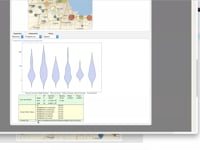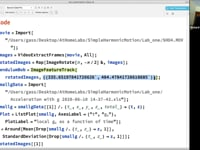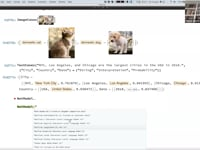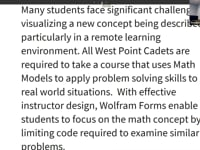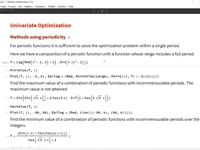Learn how »

# Using the Wolfram Cloud to Motivate Math Models via Remote Learning

CPT Timothy Newlin of West Point talks about how he uses Wolfram technology for online math courses. With effective instructor design, Wolfram forms enable students to focus on the math concept by limiting code required to examine similar problems. Highlighted here are examples including difference equations, flow networks, vector addition, matrix vector transformation and fitting function-based math models to data.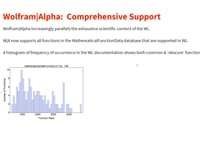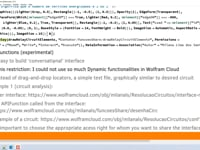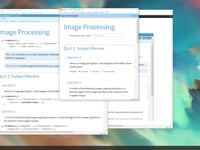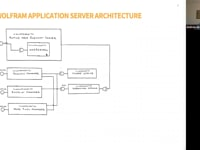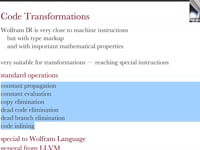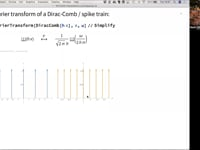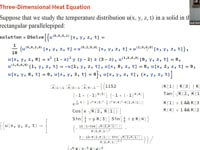The Math(s) Fix57:45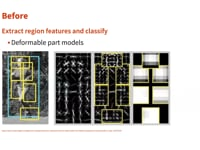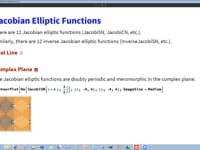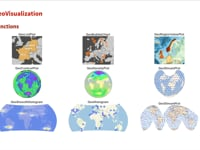Geo Visualization29:57